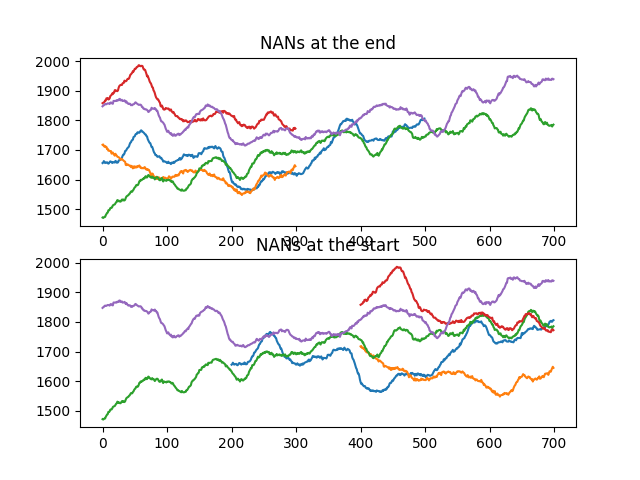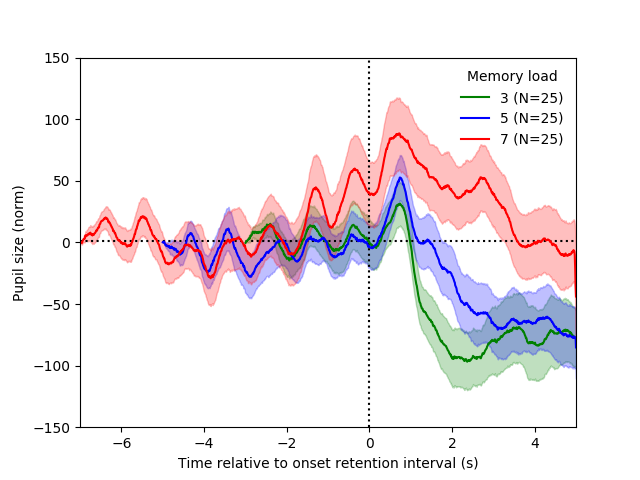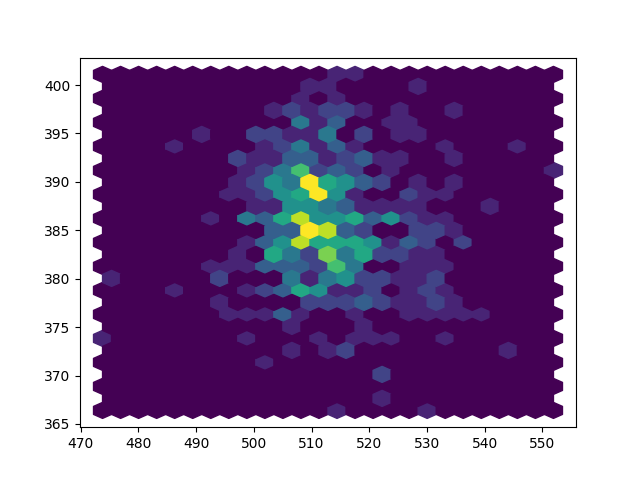# Analyzing eye-movement data

We're going to analyze pupil-size data from an auditory-working-memory experiment. This data is taken from Mathôt (2018), and you can find the data and experimental materials here.

In this experiment, the participant first hears a series of digits; we will refer to this period as the `sounds` trace. The number of digits (set size) varies: 3, 5, or 7. Next, there is a retention interval during which the participant keeps the digits in memory; we will refer to this period as the `retention` trace. Finally, the participant enters the response.

We will analyze pupil size during the `sounds` and `retention` traces as a function of set size. As reported by Kahneman and Beatty (1966), and as we will also see during this tutorial, the size of the pupil increases with set size.

This tutorial makes use of the `eyelinkparser` module, which can be installed with pip:

```pip install python-eyelinkparser
```

The data has been collected with an EyeLink 1000 eye tracker.

## Designing an experiment for easy analysis

EyeLink data files (and data files for most other eye trackers) correspond to an event log; that is, each line corresponds to some event. These events can be gaze samples, saccade onsets, user messages, etc.

For example, a `start_trial` user message followed by four gaze samples might look like this:

```MSG 451224 start_trial
451224    517.6   388.9  1691.0 ...
451225    517.5   389.1  1690.0 ...
451226    517.3   388.9  1692.0 ...
451227    517.1   388.7  1693.0 ...
```

When designing your experiment, it's important to send user messages in such a way that your analysis software, in this case `eyelinkparser`, knows how to interpret them. If you do, then data analysis will be easy, because you will not have to write a custom script to parse the data file from the ground up.

If you use OpenSesame/ PyGaze, most of these messages, with the exception of phase messages, will by default be sent in the below format automatically.

### Trials

The following messages indicate the start and end of a trial. The `trialid` argument is optional.

```start_trial [trialid]
end_trial
```

### Variables

The following message indicates a variable and a value. For example, `var response_time 645` would tell `eyelinkparser` that the variable `response_time` has the value 645 on that particular trial.

```var [name] [value]
```

### Phases

Phases are named periods of continuous data. Defining phases during the experiment is the easiest way to segment your data into different epochs for analysis.

The following messages indicate the start and end of a phase. A phase is automatically ended when a new phase is started.

```start_phase [name]
end_phase [name]
```

For each phase, four columns of type `SeriesColumn` will be created with information about fixations:

• `fixxlist_[phase name]` is a series of X coordinates
• `fixylist_[phase name]` is a series of Y coordinates
• `fixstlist_[phase name]` is a series of fixation start times
• `fixetlist_[phase name]` is a series of fixation end times

Additionally, four columns will be created with information about individual gaze samples:

• `xtrace_[phase name]` is a series of X coordinates
• `ytrace_[phase name]` is a series of Y coordinates
• `ttrace_[phase name]` is a series of time stamps
• `ptrace_[phase name]` is a series of pupil sizes

## Analyzing data

### Parsing

We first define a function to parse the EyeLink data; that is, we read the data files, which are in `.asc` text format, into a `DataMatrix` object.

We define a `get_data()` function that is decorated with `@fnc.memoize()` such that parsing is not redone unnecessarily (see memoization).

```from datamatrix import (
operations as ops,
functional as fnc,
series as srs
)

@fnc.memoize(persistent=True)
def get_data():

# The heavy lifting is done by eyelinkparser.parse()
dm = parse(
folder='data', # Folder with .asc files
traceprocessor=defaulttraceprocessor(
downsample=10 # Reduce sampling rate to 100 Hz
)
)
# To save memory, we keep only a subset of relevant columns.
dm = ops.keep_only(
dm,
dm.set_size, dm.correct, dm.ptrace_sounds, dm.ptrace_retention,
dm.fixxlist_retention, dm.fixylist_retention
)
return dm
```

Output:

```Initializing cache ...
```

We now call this function to get the data as a a `DataMatrix`. If you want to clear the cache, you can call `get_data.clear()` first.

Let's also print out the `DataMatrix` to get some idea of what our data structure looks like. As you can see, traces are stored as series, which is convenient for further analysis.

```dm = get_data()
print(dm)
```

Output:

```@cached: calling <function parse at 0x7fbdd4cb39d8>
data/awm.asc
Parsing data/awm.asc ........................................................................... (75 trials)
+----+---------+---------------------------+---------------------------+-----------------------------------+-----------------------------------+----------+
| #  | correct |     fixxlist_retention    |     fixylist_retention    |          ptrace_retention         |           ptrace_sounds           | set_size |
+----+---------+---------------------------+---------------------------+-----------------------------------+-----------------------------------+----------+
| 0  |    1    | [525.9 529.7 ... nan nan] | [382.9 379.8 ... nan nan] | [1810.  1810.3 ... 1902.9 1908.2] |    [1655.6 1657.1 ... nan nan]    |    5     |
| 1  |    1    | [528.9 527.7 ... nan nan] | [386.4 385.8 ... nan nan] | [1644.6 1648.7 ... 1675.4 1673.4] |    [1717.3 1718.5 ... nan nan]    |    3     |
| 2  |    1    | [521.4 523.  ... nan nan] | [377.3 367.9 ... nan nan] | [1787.  1791.4 ... 1719.9 1719.3] | [1471.8 1470.4 ... 1783.2 1785.3] |    7     |
| 3  |    1    | [523.2 513.9 ... nan nan] | [382.6 379.7 ... nan nan] | [1773.5 1774.8 ... 1755.7 1752.5] |    [1857.7 1859.1 ... nan nan]    |    3     |
| 4  |    1    | [522.1 531.6 ... nan nan] | [373.5 376.1 ... nan nan] | [1939.2 1939.6 ... 1801.3 1802.7] | [1847.2 1849.6 ... 1940.  1938.7] |    7     |
| 5  |    1    | [521.  526.9 ... nan nan] | [379.1 384.2 ... nan nan] | [1867.4 1867.9 ... 1765.6 1768.4] | [1615.9 1619.3 ... 1872.6 1870.6] |    7     |
| 6  |    1    | [520.8 532.5 ... nan nan] | [387.5 377.8 ... nan nan] | [1675.2 1674.1 ... 1585.  1581.4] |    [1826.5 1821.8 ... nan nan]    |    5     |
| 7  |    1    | [527.5 527.  ... nan nan] | [396.  390.3 ... nan nan] | [1766.5 1766.7 ... 1685.7 1682.2] |    [1728.6 1726.7 ... nan nan]    |    3     |
| 8  |    1    | [518.4 526.1 ... nan nan] | [391.8 388.3 ... nan nan] | [1733.3 1736.7 ... 1733.2 1730.2] | [1636.  1637.6 ... 1730.3 1729.3] |    7     |
| 9  |    0    | [528.2 541.3 ... nan nan] | [382.7 386.9 ... nan nan] | [1692.  1692.8 ... 1655.5    nan] | [1533.  1534.5 ... 1688.  1693.6] |    7     |
| 10 |    1    | [528.4 525.9 ... nan nan] | [388.3 378.2 ... nan nan] | [1602.2 1601.3 ... 1574.1 1575.9] | [1789.2 1793.1 ... 1599.6 1602.1] |    7     |
| 11 |    0    | [525.  519.7 ... nan nan] | [386.7 383.8 ... nan nan] | [1742.5 1740.1 ... 1567.1 1559.5] | [1696.8 1700.6 ... 1747.5 1746.8] |    7     |
| 12 |    1    | [503.1 514.6 ... nan nan] | [376.2 377.3 ... nan nan] | [1668.9 1668.8 ... 1556.1    nan] |    [1667.6 1667.6 ... nan nan]    |    5     |
| 13 |    1    | [517.2 511.3 ... nan nan] | [387.1 386.  ... nan nan] | [1609.5 1602.3 ... 1590.9 1584.3] | [1479.8 1477.9 ... 1614.1 1609.2] |    7     |
| 14 |    1    | [507.6 502.5 ... nan nan] | [388.4 386.2 ... nan nan] | [1475.4 1473.7 ... 1484.6 1490.7] |    [1481.9 1477.6 ... nan nan]    |    3     |
| 15 |    1    | [508.6 507.9 ... nan nan] | [387.  382.8 ... nan nan] | [1494.8 1494.2 ... 1522.9 1522.1] | [1522.4 1518.7 ... 1496.1 1495.4] |    7     |
| 16 |    1    | [498.6 509.6 ... nan nan] | [386.1 389.7 ... nan nan] | [1550.4 1550.3 ... 1390.8 1385.9] |    [1651.6 1649.1 ... nan nan]    |    3     |
| 17 |    1    | [510.2 500.9 ... nan nan] | [387.  380.8 ... nan nan] | [1557.6 1559.  ... 1532.2 1532. ] |    [1537.8 1534.  ... nan nan]    |    3     |
| 18 |    1    | [521.7 512.4 ... nan nan] | [382.8 382.5 ... nan nan] | [1523.1 1521.9 ... 1409.5 1409.6] |    [1471.8 1472.6 ... nan nan]    |    5     |
| 19 |    1    | [517.4 515.4 ... nan nan] | [386.5 380.5 ... nan nan] | [1500.1 1502.  ... 1500.5 1498.4] |    [1530.4 1531.  ... nan nan]    |    5     |
+----+---------+---------------------------+---------------------------+-----------------------------------+-----------------------------------+----------+
(+ 55 rows not shown)
```

### Preprocessing

Next, we do some preprocessing of the pupil-size data.

We are interested in two traces, `sounds` and `retention`. The length of `sounds` varies, depending on how many digits were played back. The shorter traces are padded with `nan` values at the end. We therefore apply `srs.endlock()` to move the `nan` padding to the beginning of the trace.

To get some idea of what this means, let's plot pupil size during the `sounds` trace for the first 5 trials, both with and without applying `srs.endlock()`.

```from matplotlib import pyplot
from datamatrix import series as srs

plt.figure()
plt.subplot(211)
plt.title('NANs at the end')
for pupil in dm.ptrace_sounds[:5]:
plt.plot(pupil)
plt.subplot(212)
plt.title('NANs at the start')
for pupil in srs.endlock(dm.ptrace_sounds[:5]):
plt.plot(pupil)
plt.clf()
```Figure 1. Pupil traces for individual trials during the sounds trace.

Next, we concatenate the (end-locked) `sounds` and `retention` traces, and save the result as a series called `pupil`.

```dm.pupil = srs.concatenate(
srs.endlock(dm.ptrace_sounds),
dm.ptrace_retention
)
```

We then perform baseline correction. As a baseline, we use the first two samples of the `sounds` trace. (This trace still has the `nan` padding at the end.)

```dm.pupil = srs.baseline(
series=dm.pupil,
baseline=dm.ptrace_sounds,
bl_start=0,
bl_end=2,
method='subtractive'
)
```

And we explicitly set the depth of the `pupil` trace to 1200, which given our original 1000 Hz signal, downsampled 10 ×, corresponds to 12 s.

```dm.pupil.depth = 1200
```

### Analyzing pupil size

And now we plot the pupil traces for each of the three set sizes!

```import numpy as np

def plot_series(x, s, color, label):

se = s.std / np.sqrt(len(s))
plt.fill_between(x, s.mean-se, s.mean+se, color=color, alpha=.25)
plt.plot(x, s.mean, color=color, label=label)

x = np.linspace(-7, 5, 1200)
dm3, dm5, dm7 = ops.split(dm.set_size, 3, 5, 7)

plt.figure()
plt.xlim(-7, 5)
plt.ylim(-150, 150)
plt.axvline(0, linestyle=':', color='black')
plt.axhline(1, linestyle=':', color='black')
plot_series(x, dm3.pupil, color='green', label='3 (N=%d)' % len(dm3))
plot_series(x, dm5.pupil, color='blue', label='5 (N=%d)' % len(dm5))
plot_series(x, dm7.pupil, color='red', label='7 (N=%d)' % len(dm7))
plt.ylabel('Pupil size (norm)')
plt.xlabel('Time relative to onset retention interval (s)')
plt.clf()
```

And a beautiful replication of Kahneman & Beatty (1966)!Figure 2. Pupil size as a function of set size.

### Analyzing fixations

Now let's look at fixations during the `retention` phase. To get an idea of how the data is structured, we print out the x, y coordinates of all fixations of the first two trials.

```for i, row in zip(range(2), dm):
print('Trial %d' % i)
for x, y in zip(
row.fixxlist_retention,
row.fixylist_retention
):
print('\t', x, y)
```

Output:

```Trial 0
525.9 382.9
529.7 379.8
531.2 378.7
526.2 381.9
529.1 388.7
nan nan
nan nan
nan nan
nan nan
nan nan
nan nan
nan nan
nan nan
Trial 1
528.9 386.4
527.7 385.8
530.8 383.9
528.7 381.6
530.0 379.0
nan nan
nan nan
nan nan
nan nan
nan nan
nan nan
nan nan
nan nan
```

A common way to plot fixation distributions is as a heatmap. To do this, we need to create numpy arrays from the `fixxlist_retention` and `fixylist_retention` columns. This will result in two 2D arrays, whereas `plt.hexbin()` expects two 1D arrays. So we additionally flatten the arrays.

The resulting heatmap clearly shows that fixations are clustered around the display center (512, 384), just as you would expect from an experiment in which the participant needs to maintain central fixation.

```import numpy as np

x = np.array(dm.fixxlist_retention)
y = np.array(dm.fixylist_retention)
x = x.flatten()
y = y.flatten()
plt.hexbin(x, y, gridsize=25)